# 20192217 2019-2020-2 《Python程序设计》实验二报告

## 1.实验内容

1.设计并完成一个完整的应用程序，完成加减乘除模等运算，功能多多益善。
2.考核基本语法、判定语句、循环语句、逻辑运算等知识点。

## 2. 实验过程及结果

C语言的实验给我灵感，我也设置一个给小学生出题的程序

q来实现循环退出，并统计正确与错误题目数量、以及正确率。

import random

count = 0
right = 0
while True:
a = random.randint(1, 11)
b = random.randint(1, 11)
dict = [["%d+%d=" % (a, b), a + b], ["%d-%d=" % (a, b), a - b], ["%d*%d=" % (a, b), a * b],
["%d/%d=" % (a, b), a // b], ["%d%%%d=" % (a,b), a % b]]
i = random.randint(0, 4)
num = input("%s? input your answer(除法结果只取商)(结束请输入q)" % (dict[i]))
if num == "q":
break
elif int(num) == dict[i]:
count += 1
right += 1
print("right")
else:
count += 1
print("wrong")

print("total is %d\nright nums is %d\n right rate is %d\n" % (count, right, right / count))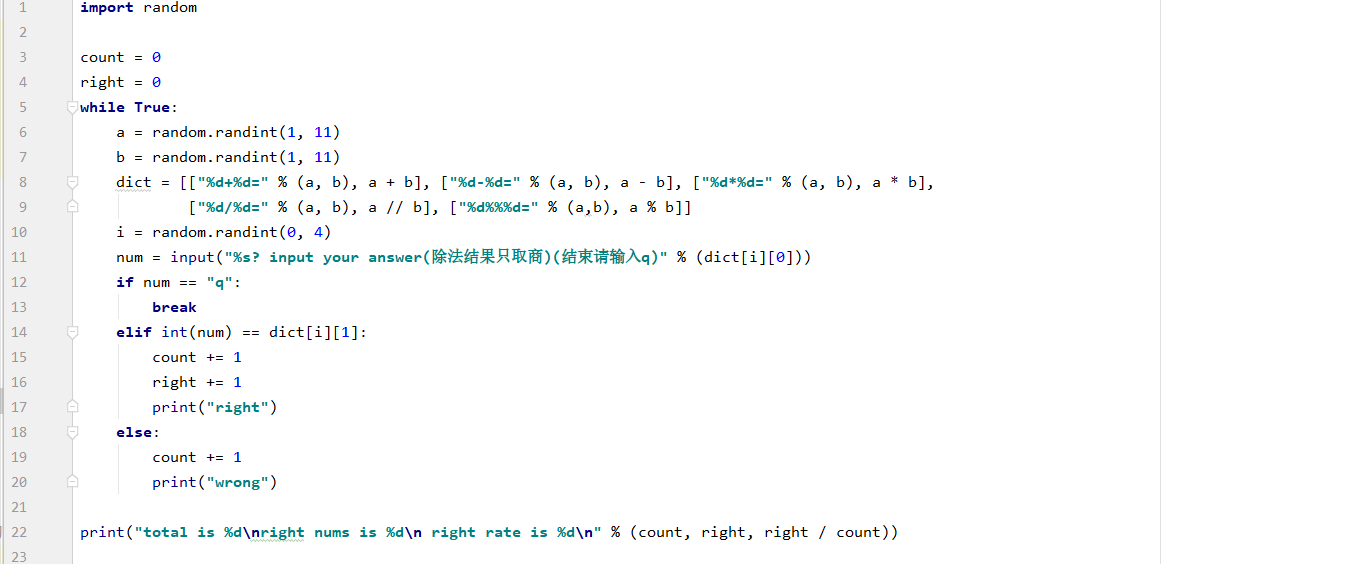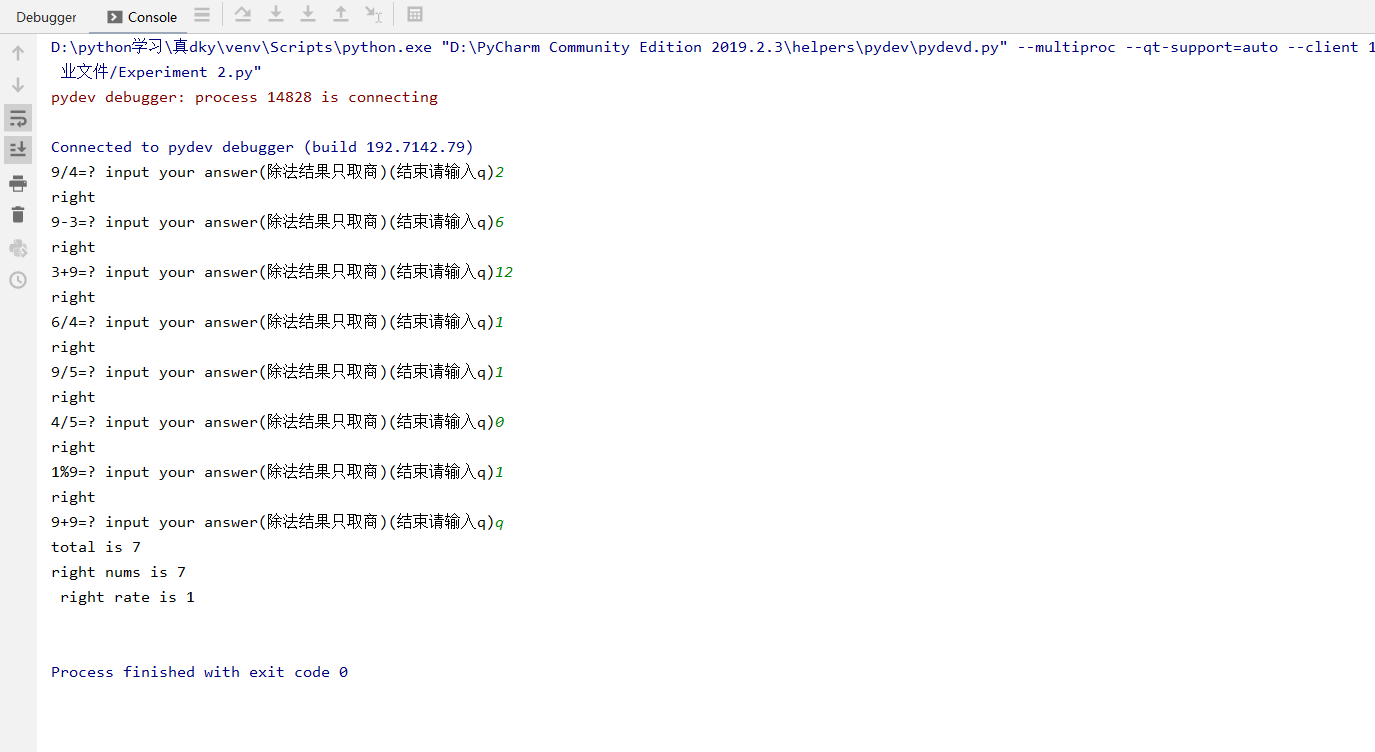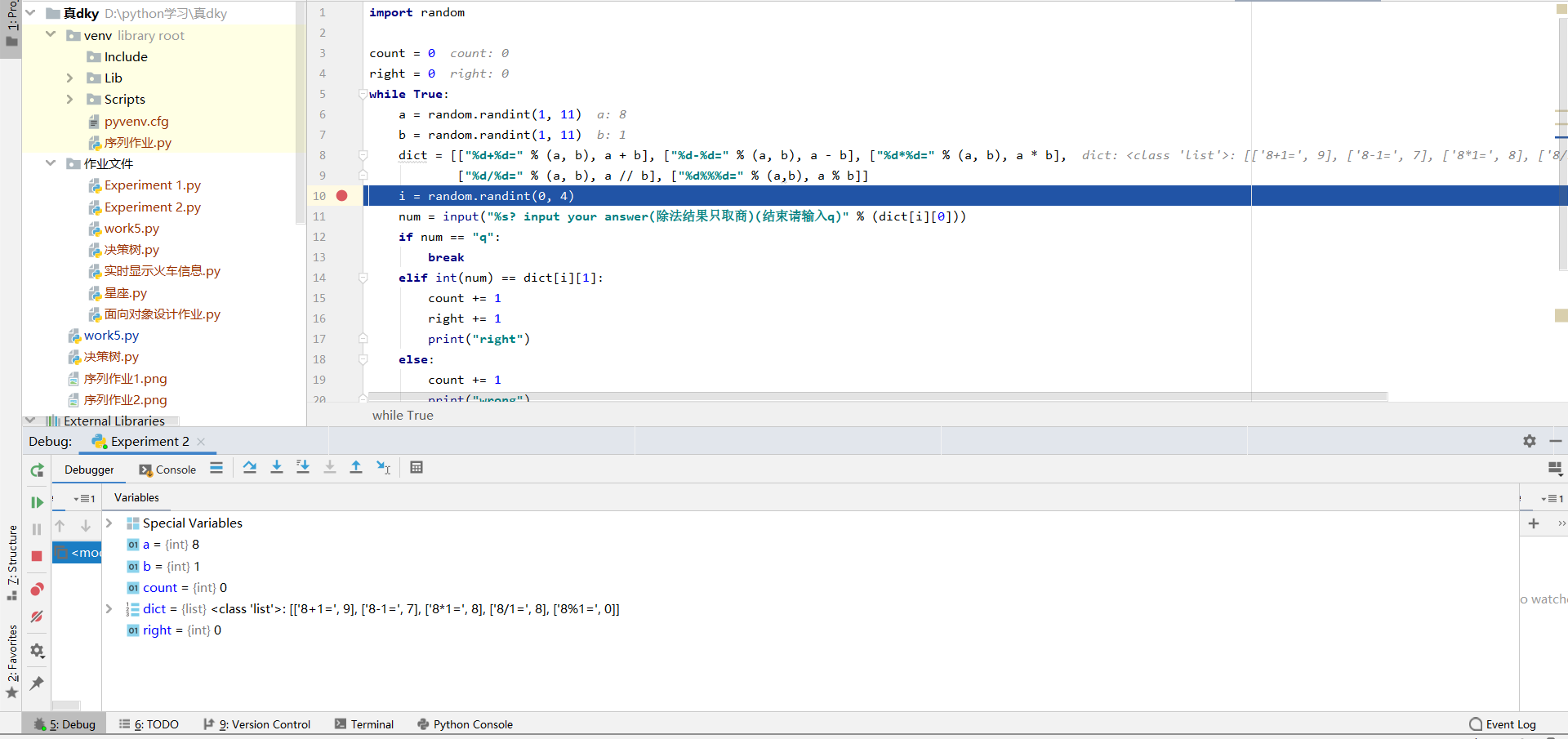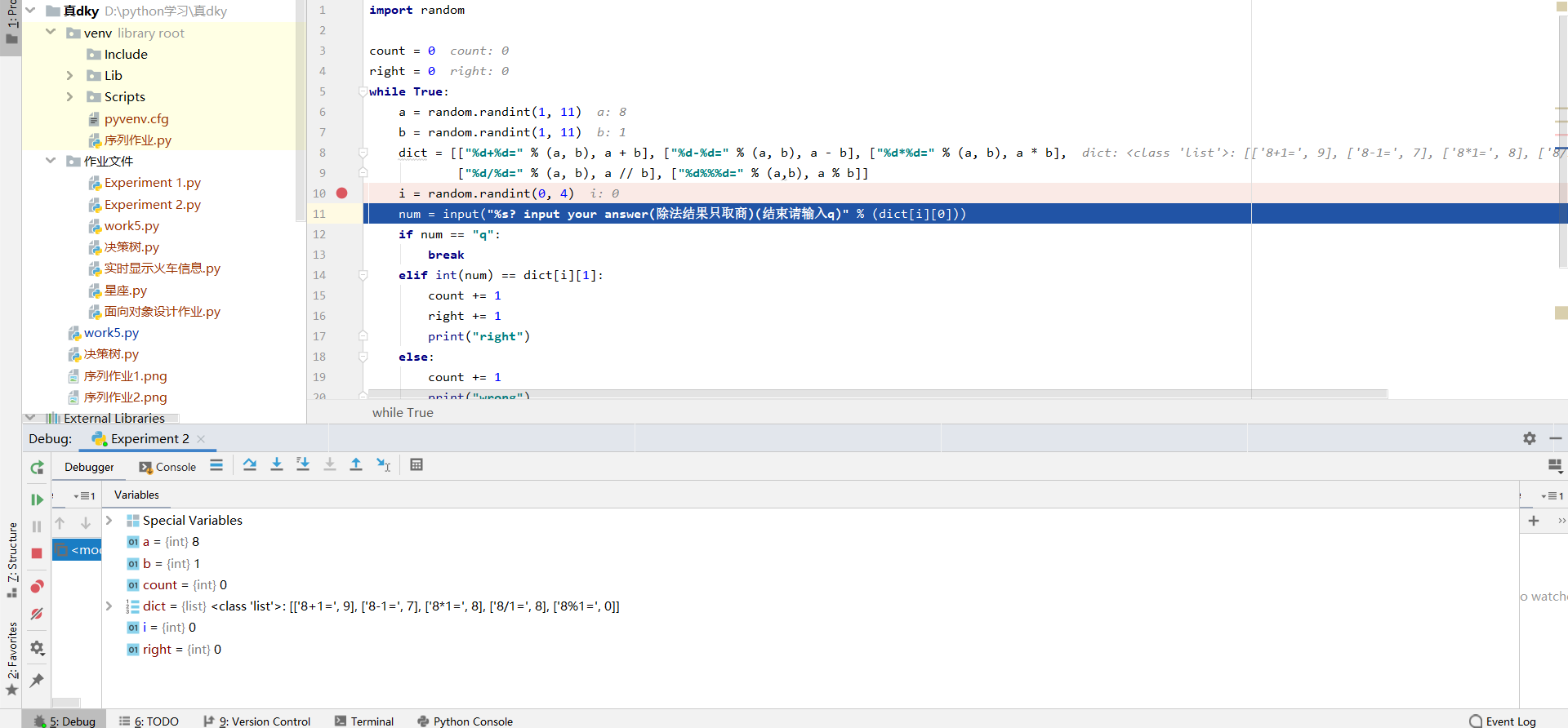## 3. 实验过程中遇到的问题和解决过程

• 问题1：一开始不知道python里的随机数怎么生成
• 问题1解决方案：通过百度很快就了解了
• 问题2：原本的代码有点冗长
• 问题2解决方案：通过百度搜索类似的代码案例研究，发现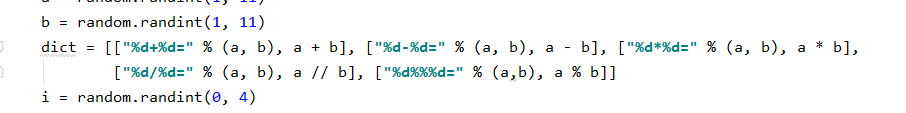通过使用列表推导式可以使代码简化了一些